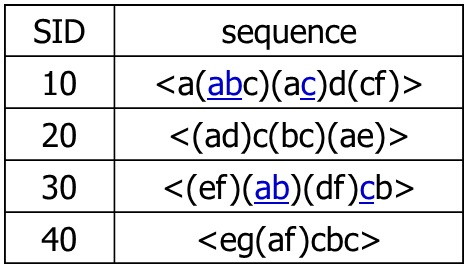July 9, 2015

## My Background

• Currently a Data Scientist at Trinity Health
• Previously a Data Analyst for a large physician practice
• MA Economics (Econometrics, Environmental Econ)

Brandon.Stange@gmail.com

## Accuracy vs. Interpretability

Maximally Accurate

• Non-Linear
• Feature Engineering/Interaction
• Ensembling

Maximally Interpretable

• Rule-Based
• Single Decision Trees
• Linear/Logistic Regression

## Association Rule Uses

• Originally used for market basket analysis
• Find sets of products that are often bought together
• Improve product arrangement
• {Beer} -> {Pizza}
• Can be used for any dataset that can be represented as a binary matrix
• Traditionally unsupervised, but can target specific outcomes
• Can target more than one outcome at once

## Algorithms and Implimentations

• Apriori
• R, Python, SQL Server, Oracle, everywhere
• Eclat
• R, Python, C, others
• FP Growth
• C, Python, mahout
• Graph Databases
• neo4j, Titan, Giraph

## Arules Package Ecosystem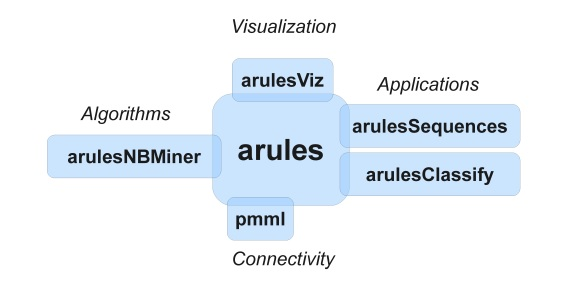## Input Data Structure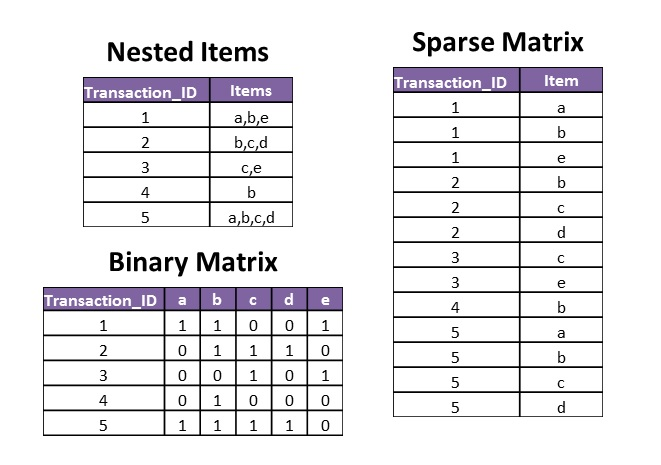## Rule Interpretation (Apriori Output)

lhs rhs support confidence lift
16 {b,c} {d} 0.4 1 2.5

RHS (Outcome) = {d}

LHS (Inputs) = {b,c} LHS occurs in 40% of total population.

RHS occurs in 100% of these transactions, which is 2.5 times the population at large.

## Apriori Function Call

apriori(data,
parameter = list(minlen=1,
support=0.05,
confidence=0.4,
maxlen=3),
appearance = list(rhs=outcomelist,
default="lhs"),
control = list(verbose=T))

## Itemset Graph Representation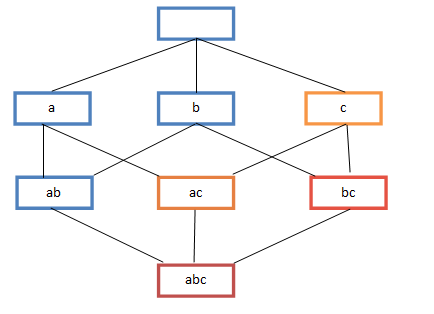## Real Data Example

490k records from New York City resturant inspections

requires readr, dplyr, tidyr, arules, arulesviz (https://data.cityofnewyork.us)

Fields of interest:

• ID, name, address, phone, etc.
• Inspection Date
• Borough
• Cuisine Description
• Violation Description
• Action

## Data Pre-Processing

1. Clean up dates and special characters
2. Apply custom violation code grouping
3. Use tidyr::gather to put data in 'long' format
4. Replace keys and measures with integer IDs
5. Convert to sparse matrix

## Pre-Processing steps 3-5 Code

## 3. Use tidyr::gather to put data in 'long' format
nycs <- nycw %>%
select(INSID, BORO, CUISINE_DESCRIPTION, VIOLATION_TYPE) %>%
gather(MEASURE, VALUE, -INSID)
nycs$MEASURE <- nycs$VALUE
nycs$VALUE <- 1 ## 4. Replace keys and measures with integer IDs ID <- unique(nycs$INSID)
ME <- unique(nycs$MEASURE) nycs$MEASURE<-match(nycs$MEASURE,ME) nycs$INSID<-match(nycs$INSID,ID) ## 5. Convert to sparse matrix sm<-sparseMatrix(i=nycs$INSID, j=nycs$MEASURE,x=nycs$VALUE,
dimnames=list(ID,ME),giveCsparse=T)

## Example Function Call

rules <- apriori(sm2,
parameter = list(minlen=1, supp=0.001, conf=0.4, maxlen=4),
appearance = list(rhs=outcomelist,
default="lhs"),
control = list(verbose=T))
outcomelist
##  "Food Handling"      "Rodents/Pests"      "Employee Practices"
##  "Temperature"        "Adminstrative"      "Poor Equipment"
##  "Food Source"
class(sm2)
##  "transactions"
## attr(,"package")
##  "arules"

## Results

30 rules were generated. The top 5 are:

lhs rhs support confidence lift
23 {BROOKLYN,Caribbean} {Rodents/Pests} 0.008 0.605 1.396
1 {Delicatessen} {Temperature} 0.010 0.599 1.353
5 {Caribbean} {Rodents/Pests} 0.016 0.570 1.315
35 {MANHATTAN,Chinese} {Temperature} 0.015 0.575 1.299
2 {Pizza/Italian} {Temperature} 0.012 0.556 1.257

## arulesViz plots

plot(rules,method="grouped")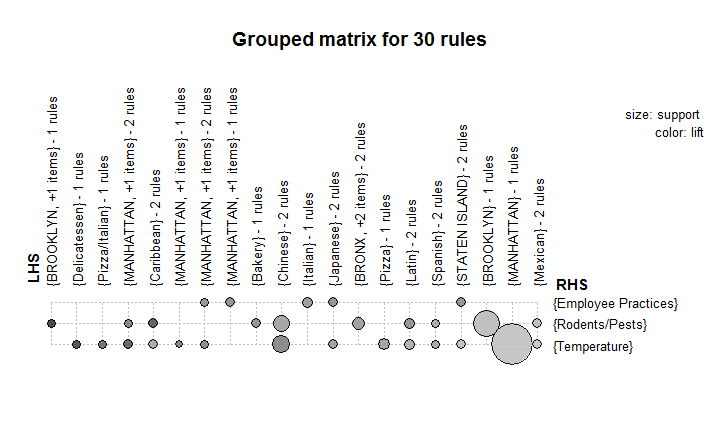## arulesViz plots contd

plot(rules,method="paracoord")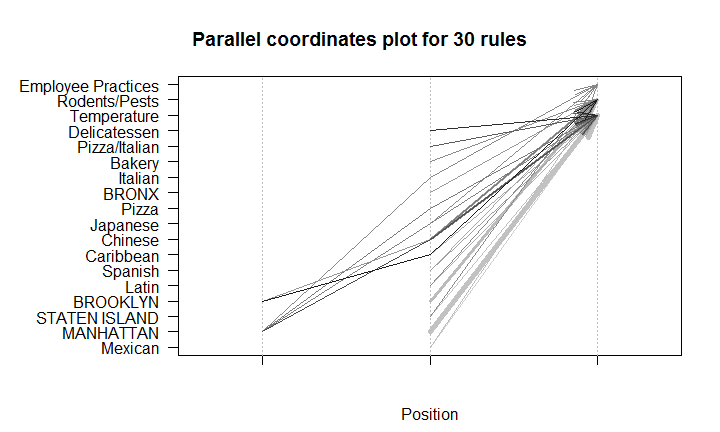## arulesViz plots contd

plot(rules,method="graph")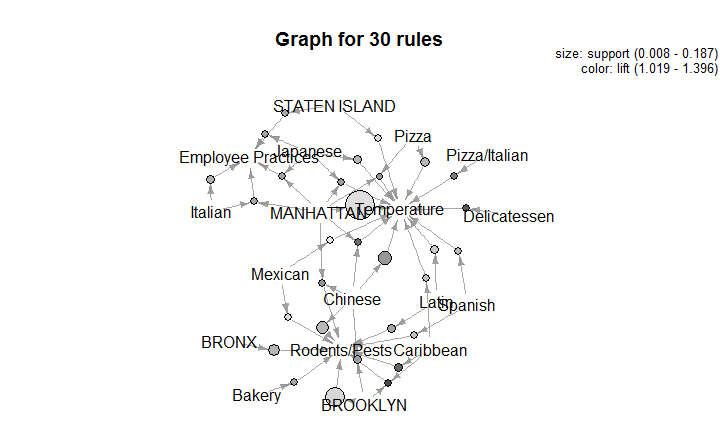## Arules Sequences

• Allows for pattern discovery in ordered sets of discrete data
• Ordered, not temporal (no measure of time between events)
• Right-censored, events after the event of interest are ignored